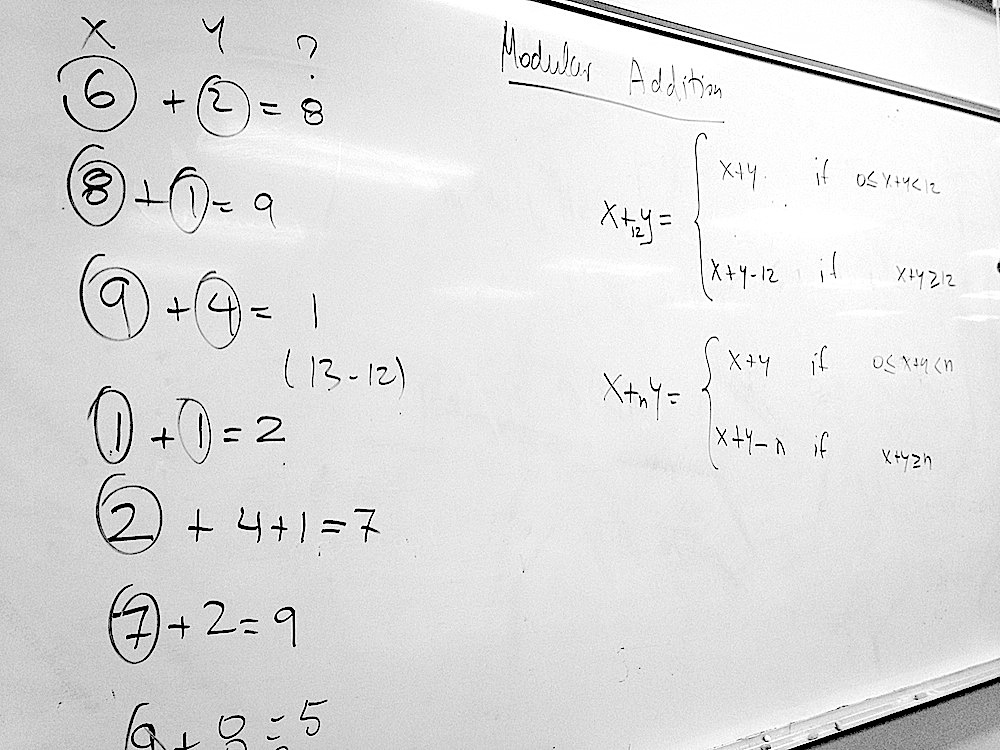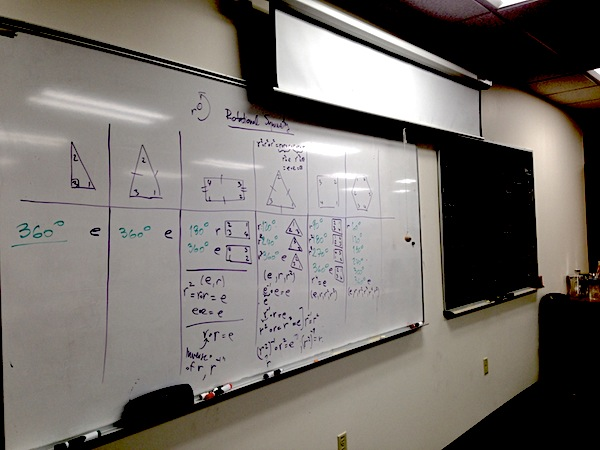## Discovering Group Theory, Day 13

On day 13, we reviewed symmetrical groups and introduced Cayley’s theorem. Students then worked out the mapping between the elements of D3 and  S3.Students explored isomorphism between D3 and S3.

## Discovering Group Theory, Day 5

On Day 5, was devoted to the discussion of  “Mathematics for Human Flourishing,” a farewell address given by Francis Su at at the end of his term as the President of the Mathematical Association of America.

Students shared their own experiences with mathematics and how those experiences shaped their attitude toward the subject.An excerpt from Francis Su’s speech.

## Discovering Group Theory, Day 4

Realizing that there was much to be gained from using now familiar cyclic groups to introduce more group properties, we postponed the exploration of dihedral groups and instead spent the day exploring.

• The review of group properties
• After reviewing the structure of particular examples of cyclic groups (C1, C2, C3, C4, and C6) students extrapolated the properties of Cn, which led us to the formal definition of cyclic groups.
• Modular Addition and (Zn,+n)
• We built on our existing knowledge of how to calculate the hour reading of a clock to motivate modular addition. Students were asked to come up with a general mathematical formula which can be used to do the calculations.
• Working in pairs, students then investigated whether (Z4,+4) forms a group by checking if it satisfies the four axioms and constructing the Cayley table.  This exercises broadened our understanding of group “multiplication,” which was originally introduced as a way to represent compounding effects of subsequent rotations of a polygon. It became clear why we have been using quotes around the word multiplication.Realizing we already know how to do modular addition.
• After comparing C4 and Z4, we introduced the idea of a group isomorphism.
• What is Not a Group? Students wanted to know more about when a set and a “product” do not form a group, so we went through a couple of examples in which one or more of the four axioms is violated (especially associativity).

## Discovering Group Theory, Day 3

On Day 3, we did two different sets of activities:

1.  We revisited the definition of a group and its properties in the context of cyclic groups, namely C1, C2, C3, C4, and C6,  encoding the rotational symmetry of polygons we played with on Day 2. Particular features of the cyclic groups prompted a natural introduction to a few new concepts and new terminology:
• Order of a group
• Order of an element of a group
• Group generators
• Group multiplication (Cayley) table, which students worked out for C3, C4, and C6 .
2. We began exploring the symmetries of a rectangle, but this time including the reflections. This allowed us study the above-listed concepts in a different context and practice carrying out group multiplication and finding inverses.

The plan was to use 2 to segue into dihedral groups, but it seems more pedagogical to spend another class or two on exploring the cyclic groups in more depth.

## Discovering Group Theory, Day 2

Topics:

• Rotational symmetry
• Identity
• Inverse
• Formal definition of a group
• Group product

On Day 2, we considered only rotations of various polygons around their geometric centers. After a systematic hands-on investigation and cataloguing of rotations which leave a polygon unchanged, we identified the identity transformations and the inverse of each rotation. The class concluded we the formal definition of a group.Cataloguing rotations of various polygons which leave them unchanged.